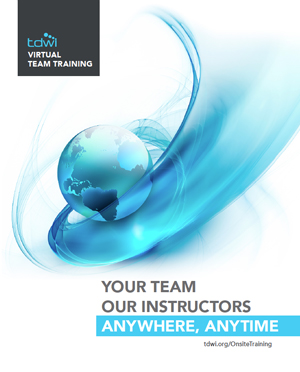## TEAM TRAINING

Our Instructors
Anywhere, Anytime

#### Machine Learning in R

Duration: One Day Course

Prerequisite: Familiarity with programming and statistics.

R is the one of the most popular machine learning tools in use today. This course focuses on introducing concepts in machine learning and applying them in practical ways using R. Topics include R data manipulation, data structures, R graphics, exploratory data analysis, basic statistics, and introduction to regression and classification modeling. Participants will complete exercises to solidify understanding and build skills with the intent of finishing the course with a toolkit that can be leveraged in building R machine learning skills.

#### You Will Learn How To

• Configure the RStudio environment and load packages
• Use R basics such as basic math, data types, vectors, and calling functions
• Use advanced data structures such as data frames, lists, and matrices
• Use R base graphic packages
• Perform exploratory data analysis
• Use R to support basic statistics, correlation, and covariance
• Use linear models such as linear regression and logistic regression
• Use models such as decision trees, random forest, and K nearest neighbor

#### Geared To

• Those interested in getting a thorough primer to machine learning and become proficient in introductory R programming

#### REQUEST INFORMATIONYvonne M. Baho Director, Enterprise Learning
Phone: 978.582.7105
Email: ybaho@tdwi.orgYvonne M. Baho